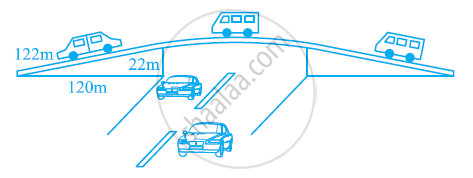# The triangular side walls of a flyover have been used for advertisements. The sides of the walls are 122m, 22m, and 120m (see the given figure). The advertisements yield an earning of Rs 5000 per m2per year. A company hired one of its walls for 3 months. How much rent did it pay? - Mathematics

The triangular side walls of a flyover have been used for advertisements. The sides of the walls are 122m, 22m, and 120m (see the given figure). The advertisements yield an earning of Rs 5000 per m2per year. A company hired one of its walls for 3 months. How much rent did it pay?#### Solution

The sides of the triangle (i.e., abc) are of 122 m, 22 m, and 120 m respectively.

Perimeter of triangle = (122 + 22 + 120) m

2s = 264 m

s = 132 m

By Heron’s formula,

"Area of triangle "=sqrt(s(s-a)(s-b)(s-c))

"Area of given triangle "=[sqrt(132(132-122)(132-22)(132-120))]m^2

=[sqrt(132(10)(110)(12))]m^2 = 1320m^2

Rent of 1 m2 area per year = Rs. 5000

Rent of 1 m2 area per month = Rs. 5000/12

Rent of 1320 m2 area for 3 months

= Rs (5000/12xx3xx1320)

= Rs (5000 × 330)

= Rs 1650000

Therefore, the company had to pay Rs 1650000.

Concept: Area of a Triangle by Heron's Formula
Is there an error in this question or solution?
Chapter 12: Heron's Formula - Exercise 12.1 [Page 202]

#### APPEARS IN

NCERT Class 9 Maths
Chapter 12 Heron's Formula
Exercise 12.1 | Q 2 | Page 202

Share